Time: 3 Hours                                                                                                     Max. Marks: 100

NOTE: There are 9 Questions in all.

·      Question 1 is compulsory and carries 20 marks. Answer to Q. 1. must be written in the space provided for it in the answer book supplied and nowhere else.

·      Out of the remaining EIGHT Questions answer any FIVE Questions. Each question carries 16 marks.

·      Any required data not explicitly given, may be suitably assumed and stated.

Q.1       Choose the correct or best alternative in the following:                                         (2x10)

a.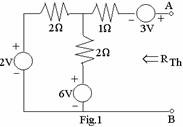The Thevenin equivalent resistance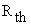for

the given network is equal to

(A)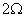.

(B)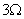.

(C)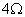.

(D)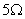.

b.      The Laplace-transformed equivalent of a given network will have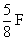capacitor replaced by

(A)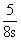.                                              (B)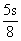.

(C)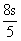.                                              (D)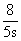.

c.   A network function contains only poles whose real-parts are zero or negative. The network is

(A)    always stable.

(B)    stable, if the j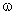-axis poles are simple.

(C)    stable, if the j-axis poles are at most of multiplicity 2

(D)    always unstable.

d.   Maximum power is delivered from a source of complex impedance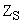to a connected load of complex impedance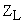when

(A)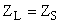(B)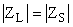(C)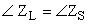(D)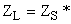e.   The admittance and impedance of the following kind of network have the same properties:

(A)     LC                                               (B)  RL

(C)  RC                                               (D)  RLC

f.    The Q-factor (or figure of merit) for an inductor in parallel with a resistance R is given by

(A)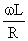.                                            (B)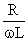.

(C)LR                                           (D)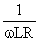.

g.   A 2-port network using z-parameter representation is said to be reciprocal if

(A)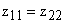.                                   (B)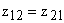.

(C)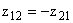.                                 (D)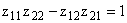.

h.    Two inductors of values L1 and L2 are coupled by a mutual inductance M. By inter connection of the two elements, one can obtain a maximum inductance of

(A)    L1+ L2 -M                                    (B) L1+ L2

(C)  L1+ L2+M                                    (D) L1+ L2+2M

i.    The expression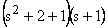is

(A)   a Butterworth polynomial.

(B)   a Chebyshev polynomial.

(C) neither Butterworth nor Chebyshev polynomial.

(D) not a polynomial at all.

j.    Both odd and even parts of a Hurwitz polynomial P(s) have roots

(A)     in the right-half of s-plane.            (B)  in the left-half of s-plane.

(C)  on the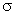-axis only.                      (D)  on the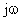-axis only.

Answer any FIVE Questions out of EIGHT Questions.

Each question carries 16 marks.

Q.2     a.   Draw the dual of the network shown in Fig.2, listing the steps involved.               (8)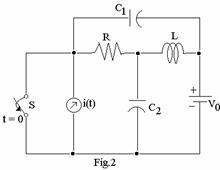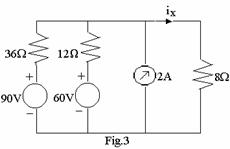b.   Using superposition theorem for the network shown in Fig.3, find the value of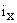.               (8)

Q.3     a.   Find the transient voltage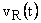40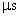after the switch S is closed at t = 0 in the network shown in Fig.4.                                                             (8)

b.   Obtain the Thevenin equivalent of the network shown in Fig.5.  Then draw the Norton’s equivalent network by source transformation.                     (8)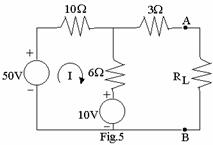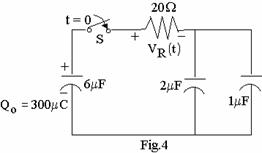Q.4     a.   Find the initial conditions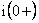and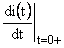for the circuit shown in Fig.6, assuming that there is no initial charge on the capacitor.  What will be the corresponding initial conditions if an inductor with zero initial current were connected in place of the capacitor?                                               (8)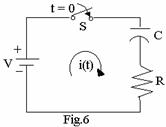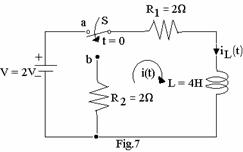b.   After steady-state current is established in the R-L circuit shown in Fig.7 with switch S in position ‘a’, the switch is moved to position ‘b’ at t = 0.  Find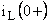and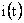for t > 0.  What will be the value of i(t) when t = 4 seconds?                                                                                                   (8)

Q.5     a.   Determine the amplitude and phase for F(j2) from the pole-zero plot in      s-plane for the network function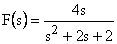.                             (8)

b.   Determine, by any method, the frequency of maximum response for the transfer functionof a single-tuned circuit. Find also the half power frequency.              (8)

Q.6     a.   For the resistive 2-port network shown in Fig.8, find v2/v1.                                  (8)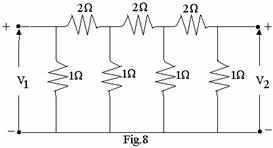b.   Show that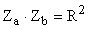holds good for both the networks given in Fig.9 if V1/I1=R.                     (8)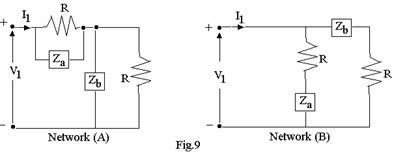Q.7     a.   Express the driving-point admittance Y(s) in the form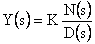, for the network shown in Fig.10. Verify that Y(s) is p.r. and  that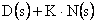is Hurwitz.                                                (8)

b.   In Fig. 11, it is required to find Y(s) to satisfy the transfer function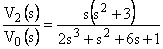Synthesise Y.                                                           (8)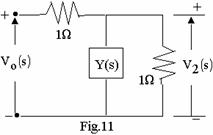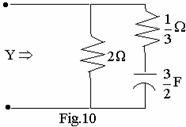Q.8     a.   Synthesise an LC network terminated in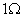, given that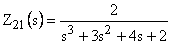.                     (8)

b.   Find the z-parameters of the network shown in Fig.12.                                       (8)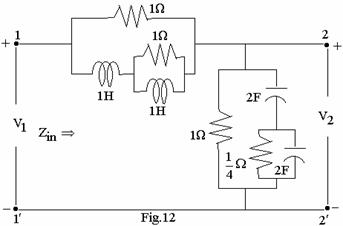Q.9     a.   Consider the system function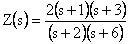.  Design:

(i)  an R-L network.

(ii) an R-C network.                                                                                         (12)

b.   Sketch the response of the magnitude function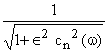where Cn

is the Chebhyshev polynomial, for n=1, 2 and 3.                                                (4)